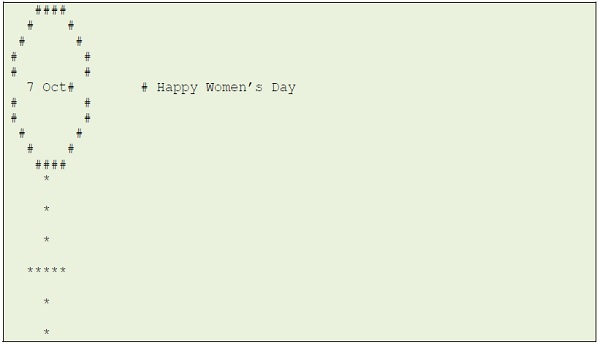# Write a program for Happy Woman’s Day in c++

The woman day which is celebrated on 7th October worldwide is carved into a c++ programming code as following;

## Example

#include <iostream>
using namespace std;
int main(){
// Initializing size of
// design
int n = 5;
// Loop to print Circle
// (Upper part of design)
// Outer loop to
// control height of
// design
for (int i = 0; i <= 2 * n; i++) {
// Inner loop to control
// width
if (i == n)
cout << " "<< "7 Oct";
for (int j = 0; j <= 2 * n; j++) {
// computing distance of
// each point from center
float center_dist = sqrt((i - n) * (i - n) + (j - n) * (j - n));
if (center_dist > n - 0.5 && center_dist < n + 0.5)
cout << "#";
else
cout << " ";
}
// Printing HappY Women's DaY
if (i == n)
cout << " "<< "Happy Women's Day";
cout << endl;
}
// Loop to print lower part
// Outer loop to control
// height
for (int i = 0; i <= n; i++) {
// Positioning pattern
// Loop for Printing
// horizontal line
if (i == (n / 2) + 1) {
for (int j = 0; j <= 2 * n; j++)
if (j >= (n - n / 2) && j <= (n + n / 2))
cout << "*";
else
cout << " ";
}
else {
for (int j = 0; j <= 2 * n; j++) {
if (j == n)
cout << "*";
else
cout << " ";
}
}
cout << endl;
}
}

## Output

The program yields the following output after the compilation of the above code as follows;